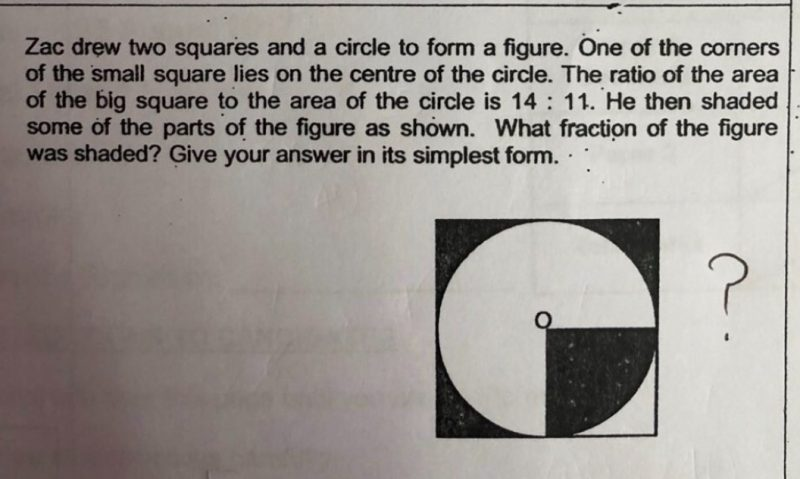# QuestionBig square : Circle = 14 : 11

Unshaded part of circle : Shaded part of circle = 3 : 1

LCM of 11 and 4 = 44

Big square : Circle = 56 : 44

Big Square : Unshaed part of circle : Shaded part of circle = 56 : 33 : 11

56 – 44 = 12

12/4 = 3

Shaded part = 3 + 3 + 3 + 11 = 20

Big square = 56

Shaded fraction = 20/56 = 5/14

0 Replies 0 Likes